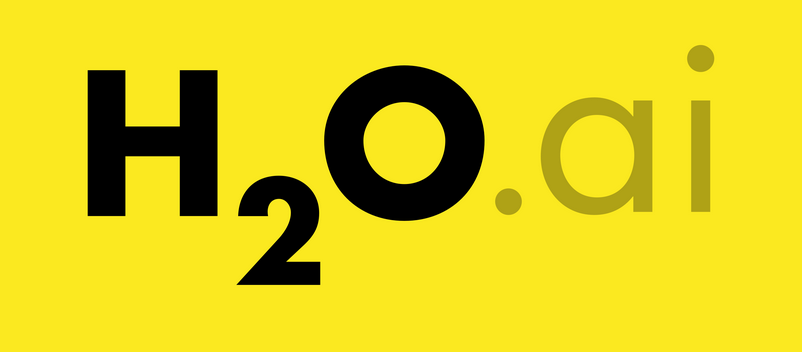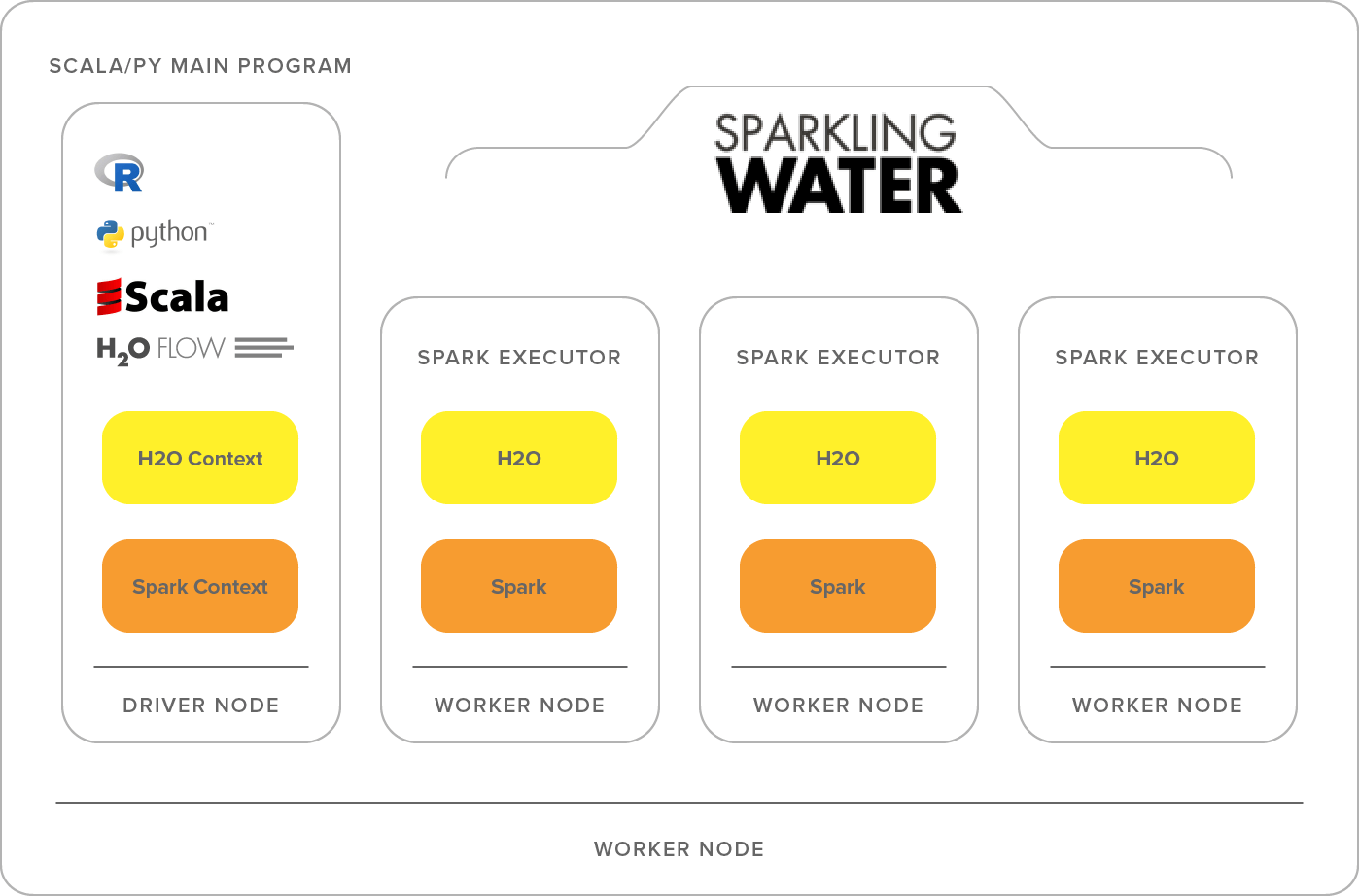# H2O AutoML

2019-12-22
Python Spark H2O## 1. What is H2O

According to their website, H2O is a fully open source, distributed in-memory machine learning platform with linear scalability. H2O supports the most widely used statistical & machine learning algorithms including gradient boosted machines, generalized linear models, deep learning and more.

It uses MapReduce to break down tasks so that it can send tasts to workers on a cluster.

H2O also has an AutoML functionality that automatically runs through all the algorithms and their hyperparameters to produce a leaderboard of the best models.

## 2. H2O AutoML

The best way to understand AutoML is by showing a practical case. As an example we will use the Higgs Challenge data. Since the preprocessing is out of the scope of this post we can directly use a copy of the preprocessed data:

### 2.1. Start H2O session

This is really straightforward:

```import h2o

h2o.init()
```

This code will init an H2O session.

### 2.2. Get data

The first step is to load the data. Then we will create a list with the names of all feature columns an another for the target. The last step is to mark the target as a `factor`. This means setting it as a target. All this can be done with:

```# Import a sample binary outcome train/test set into H2O
train = h2o.import_file("https://s3.amazonaws.com/erin-data/higgs/higgs_train_10k.csv")
test = h2o.import_file("https://s3.amazonaws.com/erin-data/higgs/higgs_test_5k.csv")

# Identify predictors and response
x = train.columns
y = "response"
x.remove(y)

# For binary classification, response should be a factor
train[y] = train[y].asfactor()
test[y] = test[y].asfactor()
```

This creates an `H2O` dataframe. You can always transform it to Pandas with `x.as_data_frame()`

### 2.3. Train

The first step is to create the `H2OAutoML` object. Since we aim to get reproducibility of the example we will set a seed. When you do so you also need to specify the maximum number of models to be trained.

```aml = H2OAutoML(max_models=20, seed=1, max_runtime_secs=training_minutes*60)
```

I also find it useful to limit the total amount of time that AutoML can spend on training.

Once the AutoML object is declared to train you only need to pass the `training_frame` and the names of both features and targets.

```aml.train(x=x, y=y, training_frame=train)
```

### 2.3. Check results

You can see the results with `lb = aml.leaderboard`. However it is more useful to see all possible information with:

```# Optionally add extra model information to the leaderboard

# Print all rows (instead of default 10 rows)
```

The total training time (for all models) of the example was limited to 2 minutes. The results are:

model_id auc logloss aucpr mean_per_class_error
StackedEnsemble_AllModels_AutoML 0.786427 0.555344 0.803633 0.319589
StackedEnsemble_BestOfFamily_AutoML 0.783762 0.557932 0.800806 0.33061
GBM_5_AutoML 0.780862 0.559708 0.79783 0.325399
GBM_1_AutoML 0.778997 0.56159 0.796523 0.326697
GBM_grid__1_AutoML_model_2 0.778615 0.591319 0.795194 0.34516
GBM_2_AutoML 0.778338 0.561527 0.79632 0.329805
GBM_3_AutoML 0.776389 0.563906 0.793284 0.328065
GBM_4_AutoML 0.770758 0.570912 0.790371 0.353743
DRF_1_AutoML 0.765151 0.580246 0.783285 0.340491
XRT_1_AutoML 0.765134 0.582172 0.783059 0.349171

## 3. H2O vs Manual ML

In order to compare the results of H2O AutoML I trained a Support Vector Classifier (SVC) and a Random Forest Classifier (RFC). Then I also did a GridSearchCV with the RandomForest to get some numbers.

The parameters for the GridSearchCV are:

```{'n_estimators': [50, 100, 150, 200], 'max_features': ['auto', 'sqrt', 'log2']}
```

And the 3 metrics analized are:

• Training time
• Prediction time
• AUC

In order to see if results could be improved I did another train with AutoML but limited to 2 hours instead of 2 minutes.

Here you can see how all models perform:

AutoML models are usally faster for predict and some of them perform better than the manual ones.

The training time with AutoML was lower than sklearn while having equal or better results.

## 4. Sparkling water

Even though H2O uses MapReduce it might be useful to integrate it with spark. To do so you only need to use Sparkling water. This will help distribute tasks to workers using Spark.To install it read the official documentation.

Once it is installed you only need to replace the H2O initalization (`h2o.init()`) for:

```from pyspark.sql import SparkSession
from pysparkling import H2OContext

spark = SparkSession.builder.appName("h2o_auto_ml").getOrCreate()
hc = H2OContext.getOrCreate(spark)
```